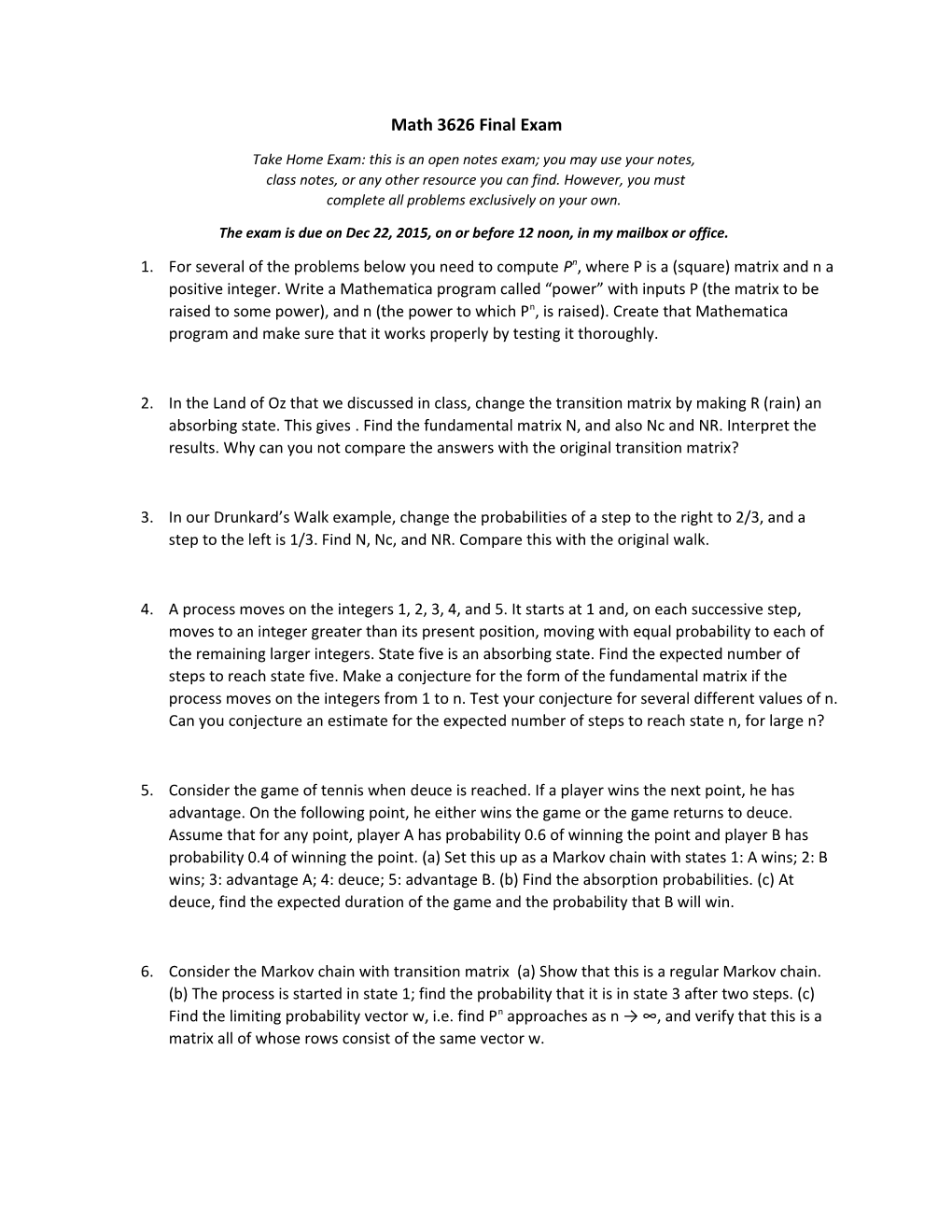# Math 3626 Final ExamMath 3626 Final Exam

Take Home Exam: this is an open notes exam; you may use your notes,
class notes, or any other resource you can find. However, you must
complete all problems exclusively on your own.

The exam is due on Dec 22, 2015, on or before 12 noon, in my mailbox or office.

1. For several of the problems below you need to compute Pn, where P is a (square) matrix and n a positive integer. Write a Mathematica program called “power” with inputs P (the matrix to be raised to some power), and n (the power to which Pn, is raised). Create that Mathematica program and make sure that it works properly by testing it thoroughly.
1. In the Land of Oz that we discussed in class, change the transition matrix by making R (rain) an absorbing state. This gives . Find the fundamental matrix N, and also Nc and NR. Interpret the results. Why can you not compare the answers with the original transition matrix?
1. In our Drunkard’s Walk example, change the probabilities of a step to the right to 2/3, and a step to the left is 1/3. Find N, Nc, and NR. Compare this with the original walk.
1. A process moves on the integers 1, 2, 3, 4, and 5. It starts at 1 and, on each successive step, moves to an integer greater than its present position, moving with equal probability to each of the remaining larger integers. State five is an absorbing state. Find the expected number of steps to reach state five. Make a conjecture for the form of the fundamental matrix if the process moves on the integers from 1 to n. Test your conjecture for several different values of n. Can you conjecture an estimate for the expected number of steps to reach state n, for large n?
1. Consider the game of tennis when deuce is reached. If a player wins the next point, he has advantage. On the following point, he either wins the game or the game returns to deuce. Assume that for any point, player A has probability 0.6 of winning the point and player B has probability 0.4 of winning the point. (a) Set this up as a Markov chain with states 1: A wins; 2: B wins; 3: advantage A; 4: deuce; 5: advantage B. (b) Find the absorption probabilities. (c) At deuce, find the expected duration of the game and the probability that B will win.
1. Consider the Markov chain with transition matrix (a) Show that this is a regular Markov chain. (b) The process is started in state 1; find the probability that it is in state 3 after two steps. (c) Find the limiting probability vector w, i.e. find Pn approaches as n → ∞, and verify that this is a matrix all of whose rows consist of the same vector w.
1. Show that the matrix does not have one fixed probability vector in the long term. In other words, find, if possible, the matrix that Pn approaches as n → ∞, and verify that it is not a matrix all of whose rows are the same. Does this contradict our very last theorem?
1. Consider our Drunkard’s Walk yet again. Assume that if the walker reaches state 0, he turns around and returns to state 1 on the next step and, similarly, if he reaches 4 he returns on the next step to state 3. Is this new chain ergodic? Is it regular? Verify that the limit Pn as n → ∞once again does not have one fixed probability vector in every row. Does this contradict our very last theorem?
1. A certain experiment is believed to be described by a two-state Markov chain with the transition matrix P, where . When the experiment is performed many times, the chain ends in state one approximately 20 percent of the time and in state two approximately 80 percent of the time. Compute a sensible numeric estimate for the unknown parameter p and explain how you found it.